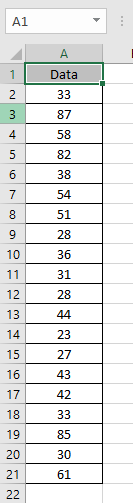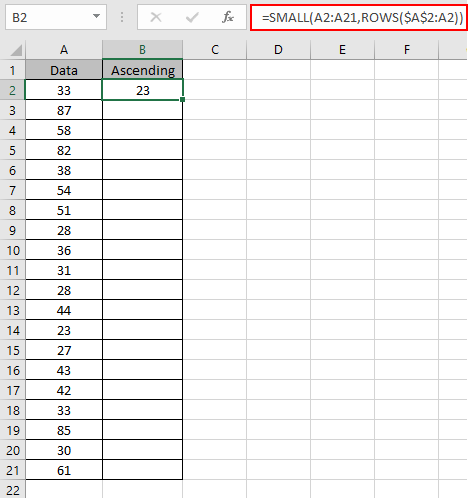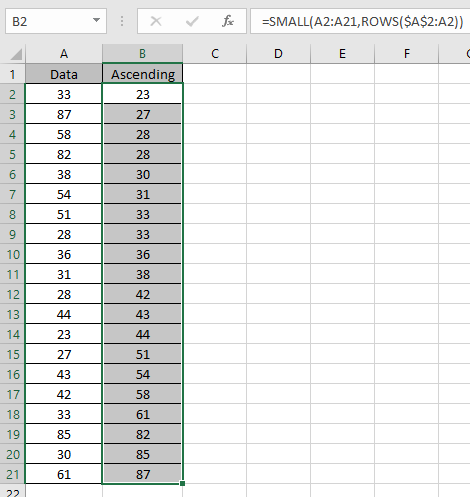# Sort numbers using Excel SMALL function

In this article, we will learn about how to Sort numbers using Excel SMALL function with ROWS function in Excel.

Sort numbers in excel using the excel functions. Excel SMALL function returns the kth smallest value from the data. We can get the data in sorted way.

The SMALL function returns the kth smallest value starting from the smallest in a range of values.
Syntax:

=SMALL (array, k)

K : k=1 return the smallest value and if k equals the number of values in an array then it returns the largest value of the data or else it returns #NUM! error. The ROWS function returns the number of rows in excel.
Syntax:

=ROWS (array)

Let’s make generic formula from the information stated above
Generic formula:

=SMALL (array, ROWS(fixed_cell : varying_cell))

Array : data of numbers
Fixed_cell : cell given as reference. For precaution take the first cell of the data as fixed cell and lock it.
varying _cell : cell reference to vary the kth value for the SMALL function.
Let’s understand this function using it in an example.Here we have a list of numbers that needed to be sort in ascending
Use the formula:

=SMALL(A2:A21,ROWS(\$A\$2:A2))

\$A\$2 : locked cell which doesn’t change when copied in excel.
A2 : unlocked cell which changes when copied to other cells.
Explanation:
The value of k here will be 1. The formula drops down to this =SMALL(A2:A21,1) as the ROWS function returns the number of rows which is 1 here..As you can see, 23 is the smallest number. Now we need this formula to generate increasing value of k for the SMALL function.

Let’s see when we use Ctrl + D shortcut key for copying the formula to other cells.As you can see we got accurate value of k for every new cell.
The trick is the value of k for the SMALL function. So here we used the ROWS function which returns increasing value of k.
Now we need to get the descending order for the same data.
So now we Use the formula:

=SMALL(A2:A21,ROWS(\$A\$2:A21))

\$A\$2 : locked cell which doesn’t change when copied in excel.
A21 : unlocked cell which changes when copied to other cells.
Explanation:
The value of k here will be 20. The formula drops down to this =SMALL(A2:A21,20) as the ROWS function returns the number of rows which is 20 here.As you can see, 87 is the largest number. Now we need this formula to generate decreasing value of k for the SMALL function.

Let’s see when we use Ctrl + D shortcut key for copying the formula to other cells.As you can see we got accurate value of k for every new cell.
The trick is the value of k for the SMALL function. So here we used the ROWS function which returns decreasing value of k.
As you can see, the SMALL function can sort numbers in both ways.
Hope you understood How to use the SMALL function in Excel. Explore more articles on Mathematical functions like SMALL and SMALL functions here. Please state your query or feedback in the comment box below.

Related Articles:

How to use the LARGE Function in Excel

How to Use RANK in Excel

Sort numbers using Excel SMALL function

Popular Articles:

The VLOOKUP Function in Excel

COUNTIF in Excel 2016

How to Use SUMIF Function in Excel

Terms and Conditions of use

The applications/code on this site are distributed as is and without warranties or liability. In no event shall the owner of the copyrights, or the authors of the applications/code be liable for any loss of profit, any problems or any damage resulting from the use or evaluation of the applications/code.